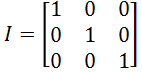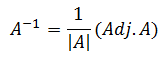Goseeko blog# What Is An Inverse Of A Matrix?

## Overview

When we talk about the inverse of a number then it is nothing but the reciprocal of that number.

The inverse of a number 5 can be written as ⅕ or 5-1

The inverse of a matrix is the same idea. If there is  a matrix A then its inverse is written as A-1.

We do not write 1/A as inverse in case of matrices.

Before moving towards the definition of inverse of a matrix, here we will understand about the identity matrix.

## Identity matrix-

A square matrix which has 1 on the diagonal and 0 on other places is called an identity matrix.

Identity matrix is denoted by ‘I’.We can write the identity matrices of order 2 by 2 or 4 by 4 etc.

Definition-

If there are two square matrices A and B of same order such that-

AB = BA = I

Then the matrix B is said to be the inverse of matrix A. or B-1=A and A is the inverse of B.

If A is a square matrix and and A-1 be its inverse then-

AA-1 = I

Where I is an identity matrix.

Note- We can find the inverse of a matrix only when it is non- singular. That means if there is a matrix  A then the inverse can be find only when |A| is non-zero.

## How do we find the inverse of a matrix?

There are many methods to find the inverse of a matrix such as – elementary transformation method (Gauss-jordan method), inverse by adjoint matrix etc.

Here we will discuss the method to find the inverse of a matrix with the help of an adjoint matrix.

Inverse of a matrix with the help of adjoint matrix-

Formula-Interested in learning about similar topics? Here are a few hand-picked blogs for you!